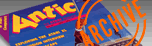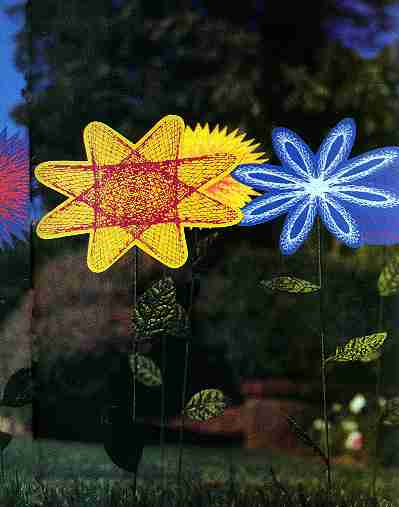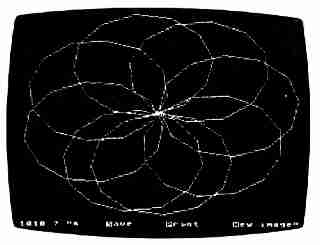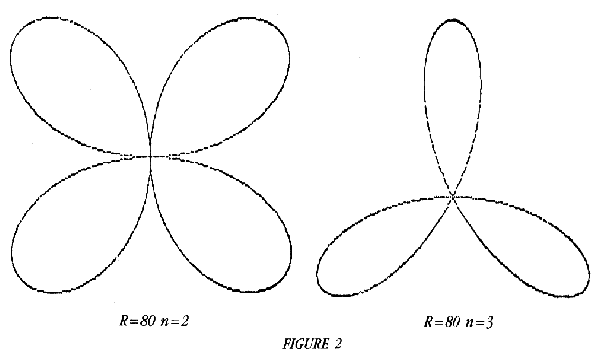`ANTIC VOL. 7, NO. 7 / NOVEMBER 1988`

# FLOWER GARDEN

INSTANT "ROSE-EQUATION"GRAPHICS

USE THE CLASSIC MATHEMATICAL
"ROSE EQUATION" TO CREATE AN ENDLESS
VARIETY OF FLOWERLIKE IMAGES FOR
SCREEN DISPLAY OR PRINTOUT.
THE BASIC PROGRAM WORKS ON 8-BIT
ATARI COMPUTERS WITH AT LEAST
32K DISK OR 24K CASSETTE.

BY ALLAN MOOSE AND MARIAN LORENZMajor advances in computer graphics have been achieved during the past 20 years and we sometimes forget that a simple line drawing program based on a mathematical function can produce pictures of unexpected beauty This article demonstrates how to grow a flower garden of graceful symmetric designs with your Atari.

The popular Cartesian coordinate system identifies a point on a plane by giving its distances from two perpendicular axes. These axes are usually labeled X and Y.

Perhaps less well known is the polar coordinate system. This system locates a point by giving its distance from the center of the coordinate system, plus an angle. For example, a fighter pilot crying out "enemy at six o'clock, nine miles out" is using a form of polar coordinates. The distance here is nine miles and the angle is 180 degrees (six o'clock).

The distance, usually labeled r for radius, is the distance from an origin, O. The angle, often labeled Theta (Θ) or Phi(Φ), is the angle between the horizontal axis and a line drawn from the origin (O) to the point (P). See Figure 1.

The rose equation, r=R*sin(n), is one of the most popular illustrations of polar coordinates.In this equation, R is a constant that determines the size of the graph and n is an integer. A graph of this equation has n petals if n is an odd integer and 2n petals if n is an even integer. Figure 2 shows the graph if R=80 for n=2 and n=3.

The program accompanying this article draws polygons inside an n-petaled rose. This program is ideal for experimentation and you will find yourself spending hours "growing flowers."

GETTING STARTED
Type in Listing 1, FLOWERS.BAS, check it with TYPO II and SAVE a copy before you RUN it. When RUN, the program will ask you to type values for N and Alpha (the angle increment). Then your Atari will draw your flower.

When it's done, type [S] to save your flower as a Micro-Painter-compatible disk file called "D:PICTURE" - the program doesn't save to cassette, sorry. Type [P] to print your flower with any Epson-compatible printer, or type [N] to erase your flower and begin a new one.HOW IT WORKS
1. Type in values for N and Alpha.

2. Next, the program initializes Count, Countstop, Delta and Deltainc. Countstop is a value that stops the program when the figure is completed. Delta is an "angle monitor." It prevents the program from drawing degenerate figures made from a single point or just a few lines. Delta insures that the ending point meets the starting point. Otherwise, the program continues drawing.

3. Now the program gets the starting point for a drawing sequence. It sets the angle Theta = Delta, computes the points Phi = π * (Theta)/180 and R = Radius * Sin(N * Phi). Finally it converts these polar coordinates (Phi, Theta) to rectangular coordinates and stores the result in Xold and Yold.

4. Next, add Alpha to Theta. If Theta is greater than or equal to 360 replace Theta by Modulo (Theta) to keep its value between 0 and 359. An explanation of Modulo is given later in the article.

5. Compute N*Theta. Reduce it by Modulo 360 and store the result in Phi. Compute R=Radius*Sin(Phi).

6. Change the polar coordinates (Theta, R) to rectangular coordinates called Xnew and Ynew.

7. Draw a line from (Xold,Yold) to (Xnew,Ynew).

8. Increment Count.

9. Check Theta. If Theta=Delta, go to step 10. Otherwise set (Xold,Yold) = (Xnew,Ynew) and go back to step 4.

10. If Count is greater than or equal to Countstop, stop plotting. Otherwise set Delta = Delta + Deltainc and go back to Step 3.

PROGRAM NOTES
The radius in steps 3 and 5 depends on your computer. For an 8-bit Atari use Radius=80.

The Modulo function (Mod) returns the remainder after a division. For example, 45 divided by 6 is 7 with a remainder of 3. The results of 45 Mod 6 would be 3. This is known as modular arithmetic. Unlike ordinary arithmetic which uses an infinite range of numbers, modular arithmetic numbers repeat after a given cycle. The program uses the Modulo function to make sure that the angles Theta and NTheta stay between 0 and 359.

EXAMPLES:
359 Mod 360 = 359
360 Mod 360 = 0
361 Mod 360 = 1

EXPERIMENTING
Observe how a drawing evolves when N remains constant while Alpha ranges from 1 to 360 degrees. For small values of N, you will find similarities in how the figures evolve as Alpha varies from 1 to 90 degrees. A smooth line drawing at small Alpha values becomes wider and more lacy as Alpha increases, until the design fills in and a squarish figure appears in the center. Continuing beyond 90 degrees up to 180 degrees will, in a sense, reverse the figure's evolution.

Also try large values of N and small values of Alpha. Interesting loop-like figures are produced with N = 92, Alpha=16 or N=206, Alpha= 28. However, to draw these loop-like figures you must change Deltainc to 1 and Countstop to 360 first.

N = 6 and Alpha = 72 draws a rotating star which evolves into a "flower" that bears no resemblance to the star which drew it.

You can also try reversing the values of N and Alpha. For example N = 200 and Alpha = 20 produces an interesting flower. N = 20 and Alpha = 200 also produces an interesting design. Not all reversals make pretty flowers, but the possibilities for creating a garden are endless.

Allan Moose and Marlan Lorenz are teachers on Long Island New York. Their mathematics-oriented programs have often appeared in Antic. Most recently was Got-A-Minute Graphics in February 1988.